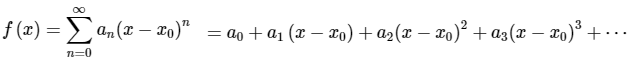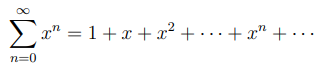# Power Series: Definition, Example & Convergence

Contents:

## What is a Power Series?

A power series, which is like a polynomial of infinite degree, can be written in a few different forms. The basic form, a summation starting with n = 0, is:A simple example is:In some situations, you may want to exclude the first term, or the first few terms (e.g., n = 0, or n = {0, 1, 2}). When this happens, the ignored terms are placed in front of the summation. As an example, this is what the series looks like if you strip out the first two terms:## Why are These Series Useful?

These series are very useful because they give us a way to represent transcendental functions (like exponential functions or logarithm functions) in a different way. This alternate form makes it easier to investigate how a function behaves for small or large changes in one of the independent variables (Cockett & Doggett, 2003).

## Power Series Convergence

These series might converge everywhere, or it might converge at a single point x. The interval where it converges is called the interval of convergence. For example, the series
1 + x + x2 + … + xn + …
is a geometric series with a fixed x-value which, for the interval of convergence (-1, 1) sums to.
Outside of (-1, 1), the series diverges.

A power series can also be complex-valued, with the form:Where (z – z0) is a series of “powers”. The convergence of a complex-valued power series is determined by the convergence of a real-valued seriesWhere w is set to w = (z – z0) (Wilde, 2006).

## Power Series Integration

Integration is a relatively simple procedure for representing an integrated function as an equivalent series summation.

Integration is particularly useful for functions that are not easily interchanged into a typical summation. The Integration step allows for the interchangeability from function to summation. A power series can be found to represent that function’s behavior, especially for one-variable functions that have continuity within a set domain of x-values.

The pursuit to find a power series for complex functions is widespread in the field of Differential Equations. Mathematicians use techniques like this to represent solution(s) for a differential equation in terms of a power series (Leavitt, J. A.).

## Proof Procedure

The integration proof for any given series needs to show that all terms within that eries are affected by integration; The series can have it’s integral evaluated for each term. Distribution of the integral state can be written as follows:

Let a generalized power series converge within a limited domain of x values (also known as Radius of Convergence). The limited domain has a length of R that acts as the radius of x values that makes the series converge.

Since the function f(x) represents the series and converges within the limited domain, f(x) is a continuous function and can be represented as separable terms.Since f(x) can be evaluated within |x| < R, we can rewrite f(x) = a0 + a1x + a2x2 +…+ anxn

The integral of both sides of the equation force the integration of each term through the distribution property of integrals.

For an example of definite integrals, the allowed interval of a general power series is restricted to the length of the x-values that converge f(x). Setting the closed interval [0, z] where [0, z] is within |x| < ℝ creates the integrated power series formula:Let z = x for simpler representation:Where C is a constant that is evaluated if given an initial value for a corresponding x value.

Since each term within f(x) can be integrated to achieve a converging value, the sum of all integrated terms represents the overall integration of a power series. This integration property within power series holds true for intervals of x that exist solely in the series’ Radius of Convergence.

Choosing a value outside this radius leads to summation results that contain added duplicates, indeterminates, and infinity itself.

## Integral Power Series Conversion

One method that integration of power series can be used is for functions aren’t recognizable from typical power series transformations. An example of such can be the following:

For F(x) = ln(2-x), Find an equivalent power series

At first, the natural log function isn’t mentioned on a list of power series equivalences. Instead, one can see that if F(x) had its derivative found, a common power series function emerges and can be worked with.

Step 1: Find the first derivative of the given function and rewrite F(x) in an integral form.Step 2: Recognize a function pattern that can be directly replaced with a common power series.Step 3: Solve the integral and organize the terms.Where C is a constant value that varies based on a chosen value for x that gets plugged in.

## Integral Trick for Power Series Integration

Power series integration can also be used to find an expansion for a given integral instead of a given function. Let’s take a different version of the example above and have it attached to a definite integral:

Find the power series expansion for G(x):Step 1: Transform the inner function (inside the integral) into a power series; You’ll be taking the integral of this function.

The inner function ln(1 + t) needs to be in power series form and in its separable terms.Step 2: Substitute in the inner function with the power series and evaluate the definite integral.Where, again, C is a constant value that is determined for a chosen x value that must be within the Radius of Convergence in the calculated series.

## Formal Power Series

A formal power series (or formal series) has the form (Tao, 2016):Where:

• a = a real number where the series is centered,
• cn = the coefficient of the series,
• c0, c1, … = a sequence of real numbers (not dependent on x).

Note that the sequence cannot depend on x in order for a series to be a formal power series. For example:

• Formal series: n! (x – 3)n
• Not a formal series: 3x (x – 3)n (the coefficient 3x depends on x).

It’s not assumed that the formal series converges (although it might), and no value is assigned to “x”. The formal series was developed to sidestep the question of convergence to make analysis easier.

## References

Henrici, P. (1988). “Definition and Algebraic Properties of Formal Series.” §1.2 in Applied and Computational Complex Analysis, Vol. 1: Power Series-Integration-Conformal Mapping-Location of Zeros. New York: Wiley, pp. 9-13, 1988.
Benestad, C. et al. (2015). P. Series, Math 121 Calculus II. Retrieved January 3, 2020 from: https://mathcs.clarku.edu/~ma121/powerseries.pdf
Cockett, M. & Doggett, G. (2003). Maths for Chemists Vol 2: P. Series, Complex Numbers and Linear Algebra (Tutorial Chemistry Texts) 1st Edition. Royal Society of Chemistry.
Leavitt, Jay A. “Methods and Applications of P. Series.” American Mathematical Society, vol. 20, 1996, pp. 46–52., https://www.ams.org/journals/mcom/1966-20-093/S0025-5718-1966-0187402-4/S0025-5718-1966-0187402-4.pdf.
Tao, T. (2016). Analysis I: Third Edition (Texts and Readings in Mathematics) 1st ed. 2016 Edition. Hindustan Book Agency.
Wilde, I. (2006). Lecture Notes on Complex Analysis. Imperial College Press.
Wilf, H. (1994). Generatingfunctionology, 2nd ed. New York: Academic Press.

CITE THIS AS:
Stephanie Glen. "Power Series: Definition, Example & Convergence" From StatisticsHowTo.com: Elementary Statistics for the rest of us! https://www.statisticshowto.com/power-series/